# 1P Exponential Example

This example appears in the Life Data Analysis Reference book.

1-Parameter Exponential Probability Plot Example

6 units are put on a life test and tested to failure. The failure times are 7, 12, 19, 29, 41, and 67 hours. Estimate the failure rate for a 1-parameter exponential distribution using the probability plotting method.

In order to plot the points for the probability plot, the appropriate reliability estimate values must be obtained. These will be equivalent to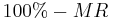since the y-axis represents the reliability and the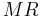values represent unreliability estimates.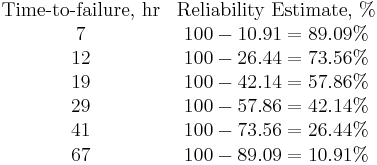Next, these points are plotted on an exponential probability plotting paper. A sample of this type of plotting paper is shown next, with the sample points in place. Notice how these points describe a line with a negative slope.

Once the points are plotted, draw the best possible straight line through these points. The time value at which this line intersects with a horizontal line drawn at the 36.8% reliability mark is the mean life, and the reciprocal of this is the failure rate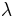. This is because at: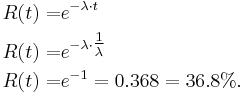The following plot shows that the best-fit line through the data points crosses the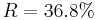line at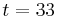hours. And because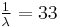hours,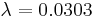failures/hour.